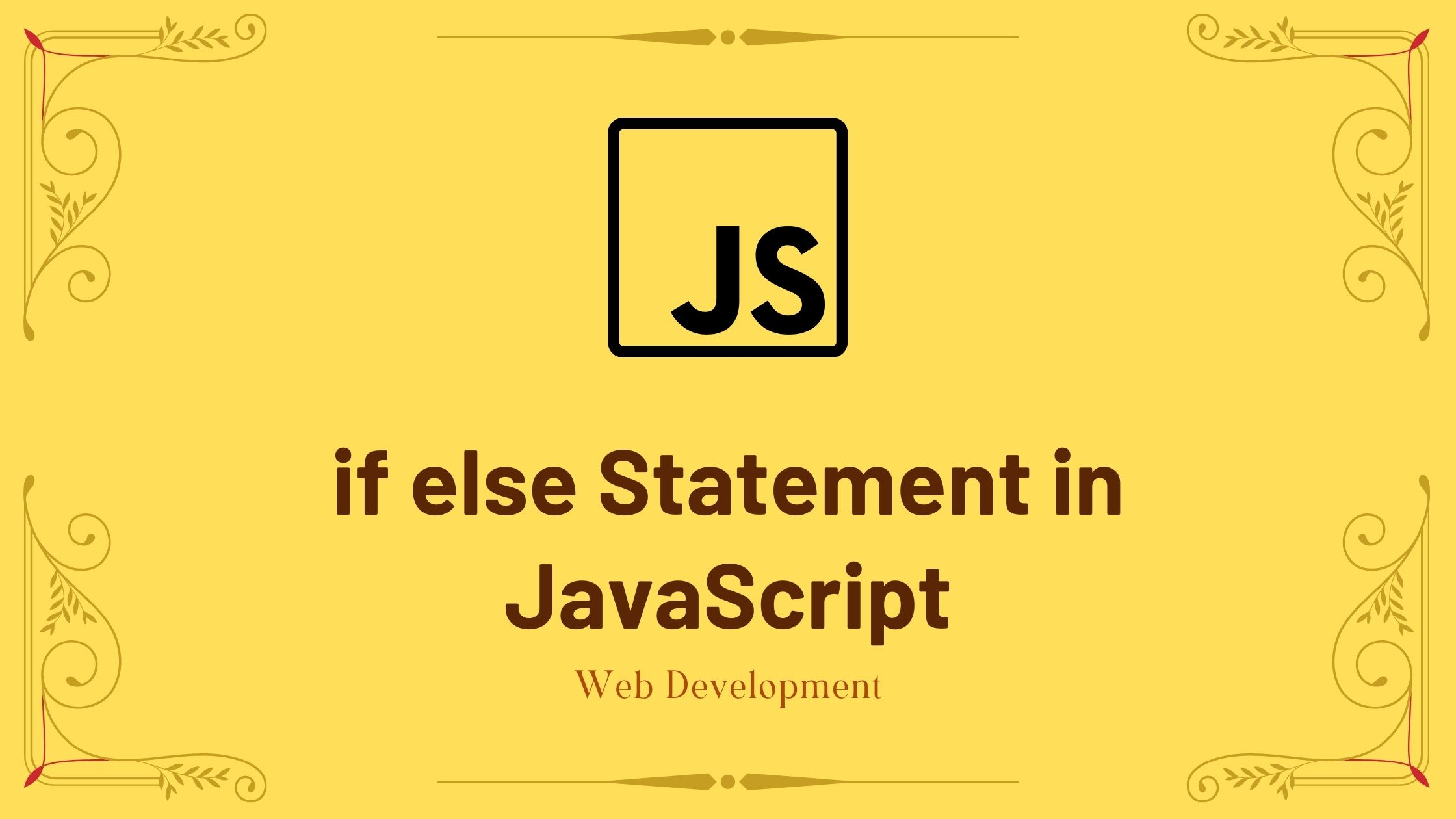## if-else Statements in JavaScript

In computer programming, there may be times when you must choose between several options when running a block of code. For example, assigning grades A, B, or C based on a student’s performance.

In such cases, you can use the JavaScript if-else statements to create a decision-making program.

There are three types of if-else statements in JavaScript.

• if statement
• if-else statement
• if-else if-else statement

Contents

## if Statements in JavaScript

if statements have the following syntax:

The condition inside the parenthesis is evaluated by the if statement ().

1. If the condition is true, the code in the body of the if statement is executed.
2. The code inside the body of if is skipped, if the condition is evaluated to false.

Note The code inside the {} of the if statement is the body of the if statement.

let’s take an example to understand if statements more easily.

For example:

Output: if we enter a positive integer

Assume the user typed in 5. The condition number > 0 evaluates to true in this case. As a result, the if statement’s body is executed.

Output: if we enter a negative number

Assume the user typed -1. The condition number > 0 evaluates to false in this case. As a result, the if statement’s body is skipped.

console.log(“The if statement is easy”); is always executed because it is outside the body of the if statement.

## if-else statement in JavaScript

An optional else clause can be added to an if statement. The if-else statement has the following syntax:

The condition inside the parenthesis is evaluated by the if-else statement.

• if it is evaluated to be true.
• the code in the body of the if statement is executed
• the code in the body of the else statement is skipped.
• if it is evaluated to be false.
• the code in the body of the else statement is executed
• the code in the body of the if statement is skipped.

let’s take an example to understand if-else statements easily.

For example:

Output: if we enter a positive integer

Assume the user typed in 5. The condition number > 0 evaluates to true in this case. As a result, the if statement’s body is executed, while the else statement’s body is skipped.

Output: if we enter a negative number

Assume the user typed -1. The condition number > 0 evaluates to false in this case. As a result, the else statement’s body is executed while the if statement’s body is skipped.

## if-else if-else statement in JavaScript

The if-else statement is used to choose between two options for executing a block of code but If you need to choose between more than two options, you can use the if-else if-else statement.

The if-else if-else statement has the following syntax:

• Code block 1 is executed, if condition1 evaluates to true.
• Condition2 is evaluated, if condition1 evaluates to false.
• Code block 2 is executed, if condition2 is true.
• Code block 3 is executed, if condition2 is false.

let’s take an example to understand if-else if-else statements better.

For example:

Output: if we enter a 0.

The first test condition number > 0 evaluates to false if the user entered 0. The second test condition number == 0, evaluates to true and the corresponding block is been executed.

### Nested if…else Statement

An if-else statement can also be used inside another if-else statement. It is known as Nested if-else statements.

let’s take an example to understand nested if-else statements better.

For example:

Output:

Assume the user typed in 5. The condition number >= 0 evaluates to true in this case, and the program is controlled by the outer if statement.

The inner if statement’s test condition, number == 0, is then evaluated. The else clause of the inner if statement is executed because it(inner if) is false.

Note: As you can see, nested if-else complicates our logic, so we should try to avoid using it as much as possible.

That’s all about the if-else statements in JavaScript.

You may like Type Conversions in JavaScript.

Hope this article will guide you to recognize the if-else statements in JavaScript that you needed and still if you have any problems or queries regarding this, post them in the comments section and we will be glad to assist you.